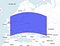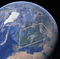# Squaring the sphere

Let’s talk about geographical bounding boxes.

Bounding boxes are easy when you are on a 2D plane. E.g. PostGIS has box2d type that describes rectangular geometry. It is usually described by low-left and up-right points. You can ask for geometry’s bounding box, or check if a geometry intersects some box, by implicitly using box2d type as geometry:

`-- PostgreSQL code, don't try in BigQuerySELECT ST_Intersects(ST_Point(2, 3),                      ST_MakeBox2D(ST_Point(1, 2), ST_Point(3, 4)))`

Things are different in BigQuery or in PostgreSQL when you use Geography type. The Earth is not flat, and Geography type works on sphere. What corresponds to bounding box on the sphere? If we take “rectangle” Geography shape, say `POLYGON((0 40, 60 40, 60 60, 0 60, 0 40))` it will look nothing like a rectangle, since Geography uses geodesic edges. Here is how it looks on a flat map:POLYGON((0 40, 60 40, 60 60, 0 60, 0 40)) on flat map

And it is not much of a rectangle on the sphere either:POLYGON((0 40, 60 40, 60 60, 0 60, 0 40)) on the globe

OK, the geodesic edges don’t make much sense for rectangular bounding box. Let’s switch back to planar map, and use Cartesian edges. It will now be a true rectangle on map, and look like this on globe:

Unfortunately, the resulting shape can no longer be described by a Geography object. The best we can do is approximate the two parallels with a lot of smaller geodesic edges that follow the parallels. BigQuery does this when we read a GeoJson geometry describing this rectangle. But the result could be a complex shape, making it useless for any practical usage. Instead, BigQuery’s `ST_IntersectsBox` function just accepts left (Westmost), low (Southmost), right (Eastmost), and high (Northmost) coordinates, like

`-- BigQuery version of the querySELECT ST_IntersectsBox(ST_GeogPoint(2, 3), 1, 2, 3, 4)`

OK, we’ve figured out what’s going on with latitudes and parallels, but what about longitudes? They are usually simple, but let’s see how one would describe bounding box of Alaska. Wikipedia says its longitudes span from 130°W to 172°E. But if we start from 130°W (Eastmost end of Alaska) and move West until we reach 172°E, we would cover most of the globe, except Alaska. We should describe it as span from 172°E to 130°W, or from 172 to -130, and we can check if a point intersects the Alaska bounding box using expression

`ST_IntersectsBox(geo, 172, 51, -130, 72)`

Note that our box looks strange — min_x coordinate (172º) is greater than max_x (-130º). But that’s OK — remember that the min_x is Westmost longitude, and max_x is Eastmost, so we are moving from 172º, cross antimeridian, and arrive at -130º. But to restore common sense and make min_x less than max_x, you can wrap the rectangle and use following expression that result in the same answer:

`--  (172 - 360) == -188ST_IntersectsBox(geo, -188, 51, -130, 72)`

or

`--  (-130 + 360) == 230ST_IntersectsBox(geo, 172, 51, 230, 72)`

All three versions describe the same bounding box:

However, don’t go too far, BigQuery still checks the bounding box is reasonable, and `max_x — min_x < 360`. E.g. BigQuery does not accept a bounding box `[172, 51, 580, 72]`: I’ve added 720 here to the second longitude, and now the semantics of the box becomes confusing — does it wrap around the globe? BigQuery rejects it for this reason.